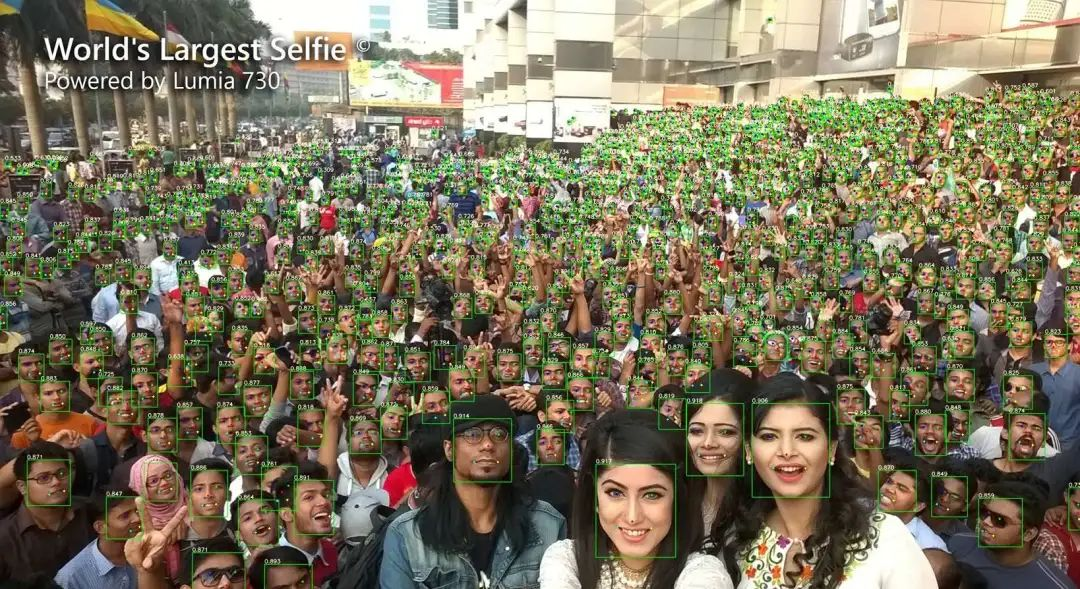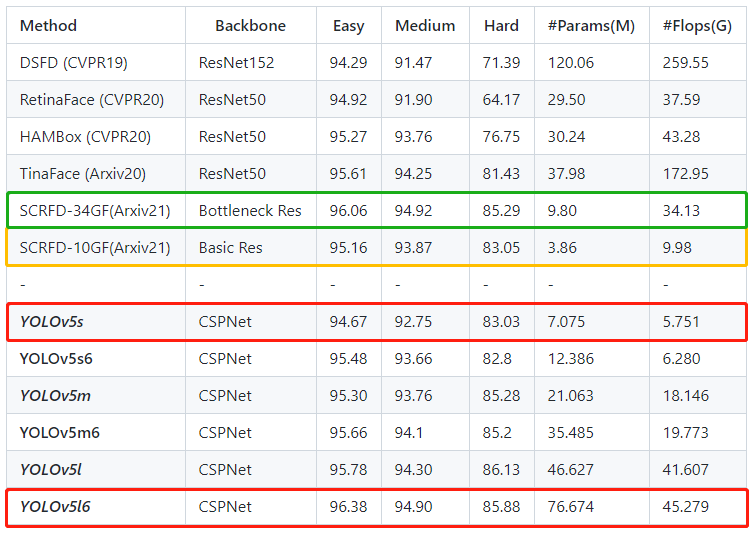Github地址：https://www.github.com/deepcam-cn/yolov5-face

## 1为什么人脸检测 = 一般检测？

• 从数据的角度来看，人脸所具有的诸如姿态、尺度、遮挡、光照以及模糊等也会出现在其他的一般检测任务之中；

• 从面部的独特属来看性，如表情和化妆，也可以对应一般检测问题中的形状变化和颜色变化。

## 2YOLOv5Face的设计目标和主要贡献

### 2.1 设计目标

YOLOv5Face针对人脸检测的对YOLOv5进行了再设计和修改，考虑到大人脸、小人脸、Landmark监督等不同的复杂性和应用。YOLOv5Face的目标是为不同的应用程序提供一个模型组合，从非常复杂的应用程序到非常简单的应用程序，以在嵌入式或移动设备上获得性能和速度的最佳权衡。

### 2.2 主要贡献

1. 重新设计了YOLOV5来作为一个人脸检测器，并称之为YOLOv5Face。对网络进行了关键的修改，以提高平均平均精度(mAP)和速度方面的性能；

2. 设计了一系列不同规模的模型，从大型模型到中型模型，再到超小模型，以满足不同应用中的需要。除了在YOLOv5中使用的Backbone外，还实现了一个基于ShuffleNetV2的Backbone，它为移动设备提供了最先进的性能和快速的速度；

3. 在WiderFace数据集上评估了YOLOv5Face模型。在VGA分辨率的图像上，几乎所有的模型都达到了SOTA性能和速度。这也证明了前面的结论，不需要重新设计一个人脸检测器，因为YOLO5就可以完成它。

## 3YOLOv5Face是如何诞生的？

### 3.1 模型架构

YOLOv5Face是以YOLOv5作为Baseline来进行改进和再设计以适应人脸检测。这里主要是检测小脸和大脸的修改。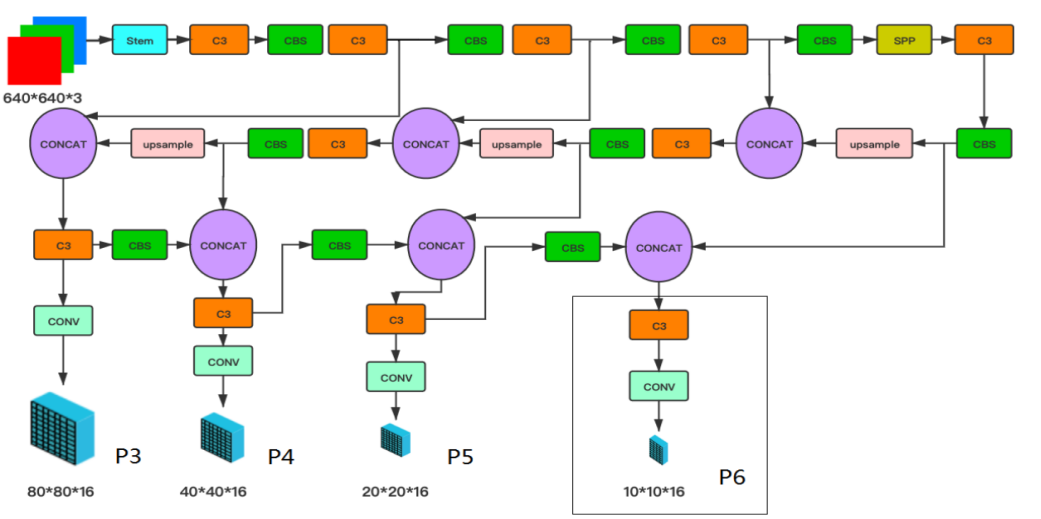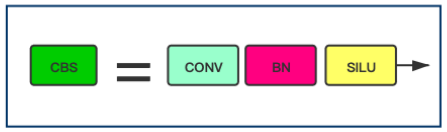``````class Conv(nn.Module):
# Standard convolution
def __init__(self, c1, c2, k=1, s=1, p=None, g=1, act=True):  # ch_in, ch_out, kernel, stride, padding, groups
super(Conv, self).__init__()
# 卷积层
self.conv = nn.Conv2d(c1, c2, k, s, autopad(k, p), groups=g, bias=False)
# BN层
self.bn = nn.BatchNorm2d(c2)
# SiLU激活层
self.act = nn.SiLU() if act is True else (act if isinstance(act, nn.Module) else nn.Identity())

def forward(self, x):
return self.act(self.bn(self.conv(x)))``````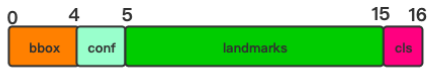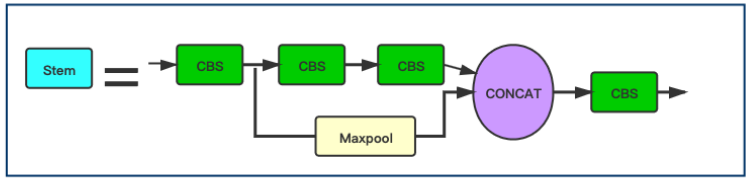``````class StemBlock(nn.Module):
def __init__(self, c1, c2, k=3, s=2, p=None, g=1, act=True):
super(StemBlock, self).__init__()
# 3×3卷积
self.stem_1 = Conv(c1, c2, k, s, p, g, act)
# 1×1卷积
self.stem_2a = Conv(c2, c2 // 2, 1, 1, 0)
# 3×3卷积
self.stem_2b = Conv(c2 // 2, c2, 3, 2, 1)
# 最大池化层
self.stem_2p = nn.MaxPool2d(kernel_size=2, stride=2, ceil_mode=True)
# 1×1卷积
self.stem_3 = Conv(c2 * 2, c2, 1, 1, 0)

def forward(self, x):
stem_1_out = self.stem_1(x)
stem_2a_out = self.stem_2a(stem_1_out)
stem_2b_out = self.stem_2b(stem_2a_out)
stem_2p_out = self.stem_2p(stem_1_out)
out = self.stem_3(torch.cat((stem_2b_out, stem_2p_out), 1))
return out``````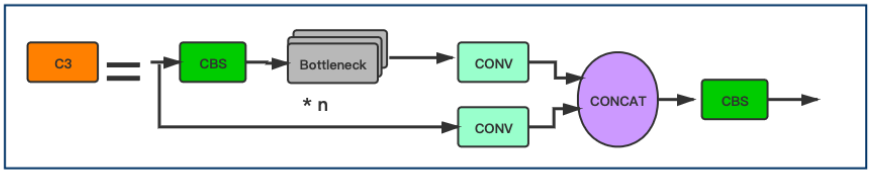``````class C3(nn.Module):
# CSP Bottleneck with 3 convolutions
def __init__(self, c1, c2, n=1, shortcut=True, g=1, e=0.5):  # ch_in, ch_out, number, shortcut, groups, expansion
super(C3, self).__init__()
c_ = int(c2 * e)  # hidden channels
self.cv1 = Conv(c1, c_, 1, 1)
self.cv2 = Conv(c1, c_, 1, 1)
self.cv3 = Conv(2 * c_, c2, 1)  # act=FReLU(c2)
self.m = nn.Sequential(*[Bottleneck(c_, c_, shortcut, g, e=1.0) for _ in range(n)])

def forward(self, x):
return self.cv3(torch.cat((self.m(self.cv1(x)), self.cv2(x)), dim=1))``````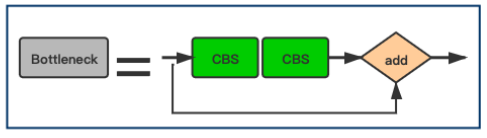``````class Bottleneck(nn.Module):
# Standard bottleneck
def __init__(self, c1, c2, shortcut=True, g=1, e=0.5):  # ch_in, ch_out, shortcut, groups, expansion
super(Bottleneck, self).__init__()
c_ = int(c2 * e)  # hidden channels
#第1个CBS模块
self.cv1 = Conv(c1, c_, 1, 1)
#第2个CBS模块
self.cv2 = Conv(c_, c2, 3, 1, g=g)
self.add = shortcut and c1 == c2

def forward(self, x):
return x + self.cv2(self.cv1(x)) if self.add else self.cv2(self.cv1(x))``````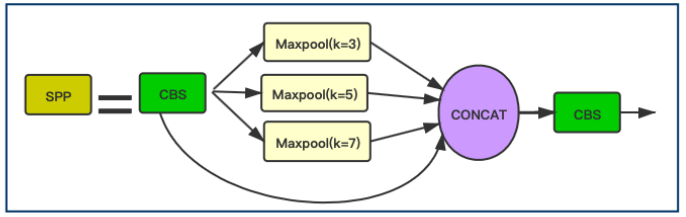``````class SPP(nn.Module):
# 这里主要是讲YOLOv5中的kernel=(5,7,13)修改为(3, 5, 7)
def __init__(self, c1, c2, k=(3, 5, 7)):
super(SPP, self).__init__()
c_ = c1 // 2  # hidden channels
# 对应第1个CBS Block
self.conv1 = Conv(c1, c_, 1, 1)
# 对应第2个 cat后的 CBS Block
self.conv2 = Conv(c_ * (len(k) + 1), c2, 1, 1)
# ModuleList=[3×3 MaxPool2d,5×5 MaxPool2d,7×7 MaxPool2d]
self.m = nn.ModuleList([nn.MaxPool2d(kernel_size=x, stride=1, padding=x // 2) for x in k])

def forward(self, x):
x = self.conv1(x)
return self.conv2(torch.cat([x] + [m(x) for m in self.m], 1))``````

### 3.2 输入端改进

YOLOv5Face作者发现一些目标检测的数据增广方法并不适合用在人脸检测中，包括上下翻转和Mosaic数据增广。删除上下翻转可以提高模型性能对小人脸进行Mosaic数据增广反而会降低模型性能，但是对中尺度和大尺度人脸进行Mosaic可以提高性能随机裁剪有助于提高性能

### 3.3 Landmark回归

Landmark是人脸的重要特征。它们可以用于人脸比对、人脸识别、面部表情分析、年龄分析等任务。传统Landmark由68个点组成。它们被简化为5点时，这5点Landmark就被广泛应用于面部识别。人脸标识的质量直接影响人脸对齐和人脸识别的质量。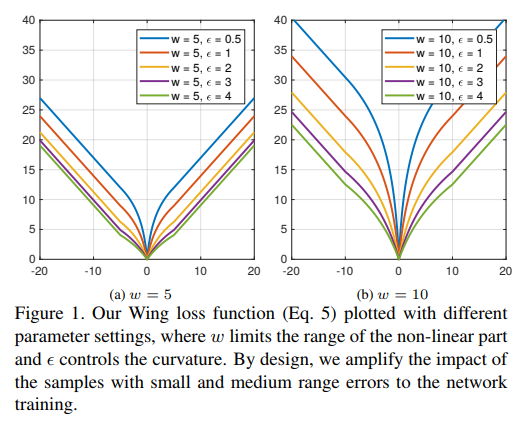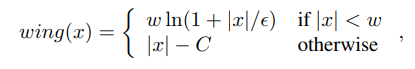w:正数w将非线性部分的范围限制在[−w,w]区间内；

:约束非线性区域的曲率，并且是一个常数，可与平滑的来连接分段的线性和非线性部分。的取值是一个很小的数值，因为它会使网络训练变得不稳定，并且会因为很小的误差导致梯度爆炸问题。

Landmark点向量与其ground truth 的损失函数为：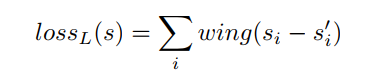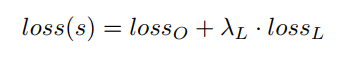landmark的获取：

``````#landmarks
lks = t[:,6:14]
lks_mask = torch.where(lks < 0, torch.full_like(lks, 0.), torch.full_like(lks, 1.0))
#应该是关键点的坐标除以anch的宽高才对，便于模型学习。使用gwh会导致不同关键点的编码不同，没有统一的参考标准
lks[:, [0, 1]] = (lks[:, [0, 1]] - gij)
lks[:, [2, 3]] = (lks[:, [2, 3]] - gij)
lks[:, [4, 5]] = (lks[:, [4, 5]] - gij)
lks[:, [6, 7]] = (lks[:, [6, 7]] - gij)``````

Wing Loss的计算如下:

``````class WingLoss(nn.Module):
def __init__(self, w=10, e=2):
super(WingLoss, self).__init__()
# https://arxiv.org/pdf/1711.06753v4.pdf   Figure 5
self.w = w
self.e = e
self.C = self.w - self.w * np.log(1 + self.w / self.e)

def forward(self, x, t, sigma=1):  #这里的x，t分别对应之后的pret，truel
weight = torch.ones_like(t) #返回一个大小为1的张量，大小与t相同
weight[torch.where(t==-1)] = 0
diff = weight * (x - t)
abs_diff = diff.abs()
flag = (abs_diff.data < self.w).float()
y = flag * self.w * torch.log(1 + abs_diff / self.e) + (1 - flag) * (abs_diff - self.C) #全是0，1
return y.sum()

class LandmarksLoss(nn.Module):
# BCEwithLogitLoss() with reduced missing label effects.
def __init__(self, alpha=1.0):
super(LandmarksLoss, self).__init__()
self.loss_fcn = WingLoss()#nn.SmoothL1Loss(reduction='sum')
self.alpha = alpha

def forward(self, pred, truel, mask): #预测的，真实的 600（原来为62*10）(推测是去掉了那些没有标注的值)
return loss / (torch.sum(mask) + 10e-14)``````

#### 分析比较L1，L2和Smooth L1损失函数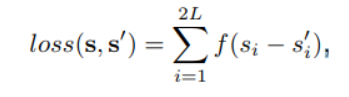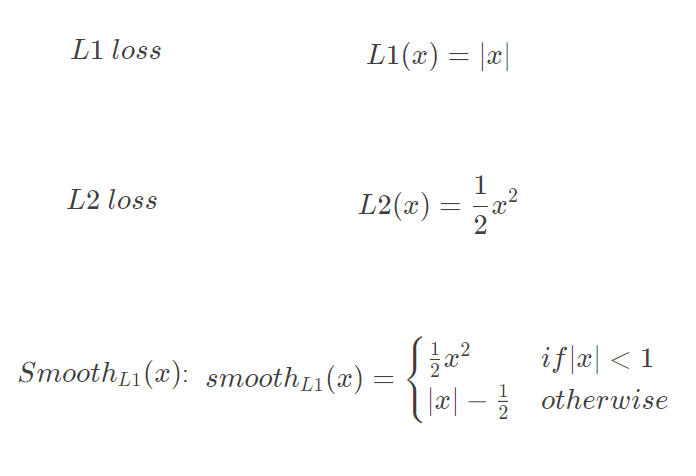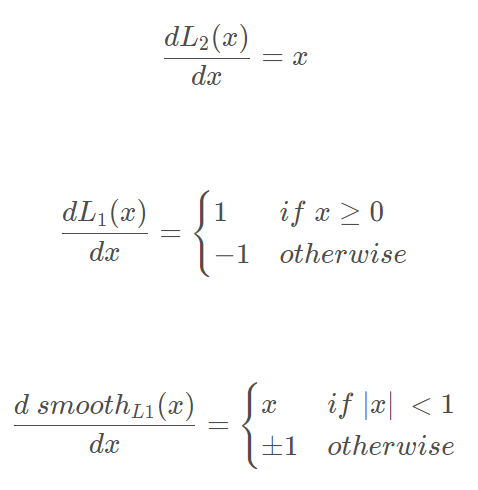L2损失函数，当x增大时L2 loss对x的导数也增大，这就导致训练初期，预测值与ground-truth差异过大时，损失函数对预测值的梯度十分大，导致训练不稳定

L1 loss的导数为常数，在训练后期，预测值与ground-truth差异很小时， 损失对预测值的导数的绝对值仍然为1，此时学习率(learning rate)如果不变，损失函数将在稳定值附近波动，难以继续收敛达到更高精度

smooth L1损失函数，在x较小时，对x的梯度也会变小，而在x很大时，对x的梯度的绝对值达到上限 1，也不会太大以至于破坏网络参数。smooth L1完美地避开了L1和L2损失的缺陷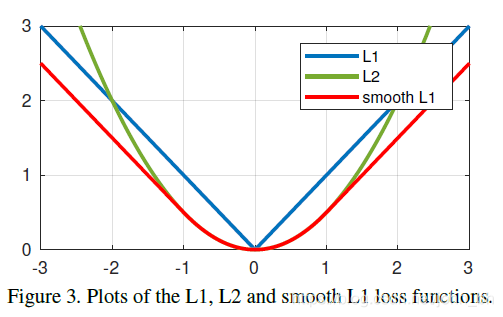#### 为什么是Wing Loss？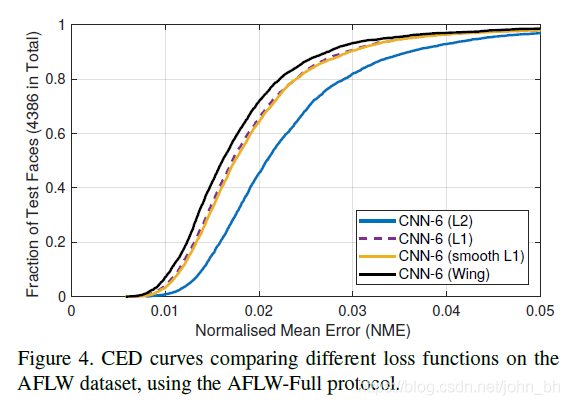### 3.4 YOLOv5Face的后处理NMS

yolov5的NMS代码如下：

``````def non_max_suppression(prediction, conf_thres=0.25, iou_thres=0.45, classes=None, agnostic=False, labels=()):
"""Performs Non-Maximum Suppression (NMS) on inference results
Returns:
detections with shape: nx6 (x1, y1, x2, y2, conf, cls)
"""

nc = prediction.shape -5  # number of classes``````

yolov5face的NMS代码如下：

``````def non_max_suppression_face(prediction, conf_thres=0.25, iou_thres=0.45, classes=None, agnostic=False, labels=()):
"""Performs Non-Maximum Suppression (NMS) on inference results
Returns:
detections with shape: nx6 (x1, y1, x2, y2, conf, cls)
"""
# 不同之处
nc = prediction.shape - 15  # number of classes``````

## 4开启训练

### 4.1 下载源码

``git clone https://github.com/deepcam-cn/yolov5-face``

### 4.3 运行train2yolo.py和val2yolo.py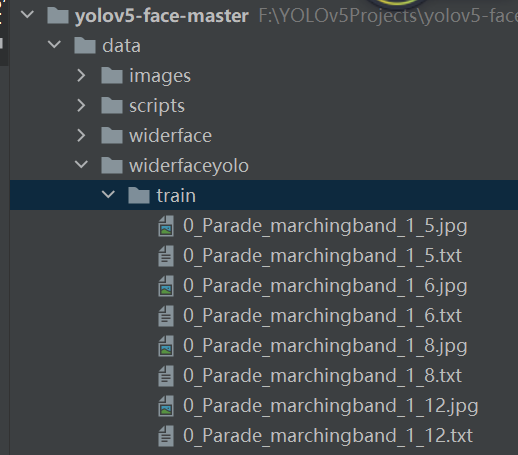### 4.4 运行train.py## 5OpenCV-C++部署

### 5.1 参数配置

``````const float anchors = { {4,5,  8,10,  13,16},
{23,29,  43,55,  73,105},
{146,217,  231,300,  335,433} };
const float stride = { 8.0, 16.0, 32.0 };
const int inpWidth = 640;
const int inpHeight = 640;
float confThreshold;
float nmsThreshold;
float objThreshold;``````

### 5.2 模型加载以及Sigmoid的定义

``````YOLO::YOLO(Net_config config)
{
cout << "Net use " << config.netname << endl;
this->confThreshold = config.confThreshold;
this->nmsThreshold = config.nmsThreshold;
this->objThreshold = config.objThreshold;
strcpy_s(this->netname, config.netname.c_str());

string modelFile = this->netname;
modelFile += "-face.onnx";
}

void YOLO::sigmoid(Mat* out, int length)
{
float* pdata = (float*)(out->data);
int i = 0;
for (i = 0; i < length; i++)
{
pdata[i] = 1.0 / (1 + expf(-pdata[i]));
}
}``````

### 5.3 后处理部分

``````if (box_score > this->objThreshold)
{
// 该部分与yolov5的保持一致
float face_score = sigmoid_x(pdata);
float cx = (sigmoid_x(pdata) * 2.f - 0.5f + j) * this->stride[n];  ///cx
float cy = (sigmoid_x(pdata) * 2.f - 0.5f + i) * this->stride[n];   ///cy
float w = powf(sigmoid_x(pdata) * 2.f, 2.f) * anchor_w;   ///w
float h = powf(sigmoid_x(pdata) * 2.f, 2.f) * anchor_h;  ///h

int left = (cx - 0.5*w)*ratiow;
int top = (cy - 0.5*h)*ratioh;

confidences.push_back(face_score);
boxes.push_back(Rect(left, top, (int)(w*ratiow), (int)(h*ratioh)));
// landmark的处理
vector<int> landmark(10);
for (k = 5; k < 15; k+=2)
{
const int ind = k - 5;
landmark[ind] = (int)(pdata[k] * anchor_w + j * this->stride[n])*ratiow;
landmark[ind + 1] = (int)(pdata[k + 1] * anchor_h + i * this->stride[n])*ratioh;
}
landmarks.push_back(landmark);
//}
}``````

## 6参考

.https://github.com/hpc203/yolov5-face-landmarks-opencv-v2
.https://github.com/deepcam-cn/yolov5-face
.YOLO5Face: Why Reinventing a Face Detector
.https://zhuanlan.zhihu.com/p/375966269``````下载1：OpenCV-Contrib扩展模块中文版教程